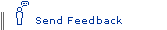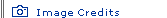Working with Infinity: A Mathematical Perspective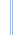Infinite Secrets homepage

For mathematicians, infinity means something completely different than for philosophers. It's not something vague and unapproachable, but rather something with a precise definition that lies at the core of modern mathematics. To explain how this eminently practical form of infinity evolved over time, and to translate for the layman how mathematicians think of it, we approached Stanford University classics historian Reviel Netz. A scholar who discovered that, contrary to belief, the ancient Greeks, through the work of Archimedes, had actually toyed with infinitely large sets, Netz knows a thing or two about mathematical infinity. For a philosophical take on the subject, see Contemplating Infinity.Defining infinityNOVA: How do mathematicians define infinity?

Netz: Something which is equal to some of its parts. That's really the technical definition.

NOVA: Is there a difference between the mathematical concept of infinity and infinity in terms, say, of space or time—the philosophical concept?

Netz: That's the curious thing. Infinity became a really clear and well-defined quantity mathematically in the late 19th century, which it wasn't before and which makes it rather different from what we ordinarily talk about when we talk about infinity, namely, about something very, very big. In mathematics nowadays, when we think about infinity, we think about a set whose properties are different from those of ordinary sets.

NOVA: Can you explain?

Netz: Well, the defining property of infinity today is that a set's cardinality [the number of elements in a given mathematical set] is equal to the cardinality of some real subset of that set. The thread that links the technical notion of infinity and the more popular/philosophical notion of infinity is the sense that infinity's hugeness being beyond reach endows it with certain paradoxical properties.

A standard example would be what was taken to be something rather paradoxical, the fact that you can take, say, the number 1 and correlate it with the number 2, take the number 2 and correlate it with the number 4, take the number 3 and correlate it with the number 6. In this way you can gradually, step by step, correlate all integer numbers (1, 2, 3, 4, etc.) with all even numbers (2, 4, 6, 8, etc.). And that's funny, because this would imply at first glance that the number of integers is equal to the number of even integers. That's the paradoxical property of infinity.An ancient discomfortNOVA: Was infinity's paradoxical nature what made the ancient Greeks so uncomfortable with it?

Netz: Yes, because of its paradoxical consequences. The paradoxical property I just mentioned is the one I start with, because it's the one that came to be the cornerstone for the contemporary treatment of infinity. However, there are other problems that would perhaps be more relevant to the Greek thinking about it.“How will you ever get from Point A to Point B if there are infinitely many things along the way?”For instance, are we going to say that a line is made of infinitely many points? This would suggest that the gradual addition of things of one kind (points) gives rise ultimately to something of a different kind altogether (a line). How do you make this leap over dimensionality? How do you get from something that has no dimension at all (a point) to something that has a real dimension (a line)? In the same way, how do you get from lines to surface? At which stage of the addition will you get there? Infinity is troublesome because it seems to imply that once you can add something up infinitely many times you can actually change something from one kind to a totally different kind. That's one kind of problem.

NOVA: What's another?

Netz: Well, infinity is also problematic because it seems to be more than anything, and for this reason it is something that you will never reach. That's the famous paradox. How will you ever get from Point A to Point B if there are infinitely many things along the way? To get from A to B, you would first have to reach half the way. But to reach half the way, you'd first have to reach half of half the way, and then to get there, you'd have to reach half of the half of the half, and so on. It would appear that you would never be able even to make a start. There are always stages along the way that you haven't reached. If things can be divided into infinitely many stretches along the way, then there is simply no way you will ever cross from one point to another.

Basically, then, the problem seems to be that whatever we're doing getting from one place to another, counting things, doing things, we're doing finite things. We're running a stadium track in two minutes; we're counting, let's say, within the range of a single book; we're making a computation on a piece of paper. We've got something finite to work with. How can anything finite encompass within it something that is infinite in character? So this seems to be another major problem.

And then there is something that is culturally specific. In the Greek context, especially in the Greek mathematical context, there was an enormous interest in trying to find ratios, harmonies, proportions that govern things. It so happens that the ratio of 2 to 3 is correlated in music with the fifth, the ratio of 3 to 4 is correlated with the fourth, the ratio of 1 to 2 is correlated with an octave. Archimedes was interested in the fact that a cylinder is exactly 3 to 2 relative to the sphere that it encompasses. A parabola is precisely 4 to 3 relative to the triangle that it encompasses. There is a sense that permeated Greek thinking in general that in order to understand things you should find the precise integer numbers that govern them.

NOVA: But infinity involves an infinity of numbers that aren't integers.

Netz: Right. If you're allowing infinity in, then you're not going to have nice integer numbers that govern the relationships. If you allow numbers to be infinitely precise, if you allow them to differ from one another in infinitesimally small amounts, then you get to the point where, for instance, there are two objects—the side of a square and its diagonal, say—and the ratio cannot be given in integer terms. The ratio is problematic; the ratio cannot be defined, because it is infinitesimally given.

The Greeks accepted that you can't actually find two integers whose ratio is the same as the ratio of the side and a diagonal in a square, or the ratio of the perimeter and diameter in a circle. But this is somehow not nice, not what you want to have. For this reason, infinity is somehow not the thing that you want to have mathematically.Of calculus and CantorNOVA: This discomfort with infinity lasted until the invention of the calculus. How did that change things?

Netz: What happens with the calculus is that you find ways to calculate infinitely long series. I've mentioned that in order to get from Point A to Point B, you first have to cross half the way, then half of the half, which is a quarter, then half of the half of the half, which is an eighth. This seems paradoxical, because it looks like we can define it this way: Let's have a series, and the series is 1/2 plus 1/4 plus 1/8, etc., going on to infinity. It would appear that the series that has infinitely many terms should be infinitely large.“What appears to be a quaint, paradoxical realm — the realm of infinite magnitude — is actually very practical.”What you find with the calculus is that there are ways of dealing with infinitely large objects in some ways that are still finite in other ways. There are ways of calculating with infinitely large objects. To start with, this was done on a rather intuitive basis. It was just a series of observations that things could be done and seem to work properly. It was only in the 19th century that precise techniques for dealing with infinitely large magnitudes emerged.

They found that once you allow yourself to do those things in practice, then you can do as a matter of calculation—real calculation with numbers—things that before you'd done purely by operating with geometrical configurations. You can think not in the qualitative terms of geometrical configurations, but in the quantitative terms of dealing with numbers and series of numbers. That's very powerful, because numbers are very powerful. They are precise and manageable in ways that geometrical configurations are not. Essentially, then, you now had a tool that is much more useful for science.

So what appears to be a quaint, paradoxical realm—the realm of infinite magnitude—is actually very practical. It's something that allows you to extend operations with numbers to any domain whatsoever. That's what happened from the 17th century onward.

NOVA: How did Georg Cantor's set theory refine mathematicians' thinking about infinity?

Netz: Well, the essence of the calculus is that you deal with infinitely large objects. But you never had to define infinity itself, and you never had to worry about the nature of infinity, primarily because you always dealt with the very same kind of infinity—roughly speaking, the infinity of points making up a line, the infinity of all the real numbers between, let's say, 0 and 1. That's the type of thing they were worried about in the calculus from the 17th century to the 19th century.

But they didn't think about what infinity is, because for one thing they didn't think about what a set is. What is a set? And then what would be the difference between a finite and an infinite set? This is something that Cantor did in the late 19th century. Cantor developed the notion of a set, and the notion of an infinite set, a set that has infinitely many objects.

Then many, many curious things turned up. We found that the paradoxical properties of infinite sets can actually be used to define them, and even more striking—and something that is slightly technical, and for this reason perhaps not something that I can show you, but you'll have to take for granted—even more curious is that the fact that you find that once you have an infinity, an infinite set, you can create another infinite set that is bigger than the infinite set you started with. You can have two infinite sets, one of them being bigger than the other. Actually, because the operation is recursive, you can take a set and create a set bigger than itself, so you can have an entire sequence of infinity. There are infinitely many infinities, stretching all the way up.

This, of course, gave rise to a fascinating field of operating with infinite numbers, which, in fact, are richer and more interesting than finite numbers are. That's the field opened up by Cantor.Infinity todayNOVA: So is infinity an active field of study for mathematicians today?

Netz: Oh, infinity is what mathematics is about. Infinity always was what mathematics is about. It's just that there are many different ways of dealing with it. In the Greek context, you dealt with situations that give rise to infinity by transforming them into geometrical representations. So, for example, you effectively dealt with how many lines it takes to fill a certain rectangle by looking at various geometrical configurations. You never called infinity by its name (with a few exceptions, including one important one discovered for Archimedes recently). [Editor's note: While reading the Archimedes palimpsest, a 10th-century copy of Archimedes' treatise The Method of Mechanical Theorems, Prof. Netz and Japanese colleague Ken Saito found that the Greek mathematician had compared two infinitely large sets and noted that they had an equal number of members. No other surviving Greek mathematics text does that.]“To do anything with the physical world — that is, physics, chemistry — you have to use the tools of infinity.”In the Scientific Revolution, you did those things with the recognition that you're dealing with infinity, and from the 19th century onwards you began dealing with actual infinite sets—a mathematics of infinity. The kinds of infinity that are allowed into the game define the kind of mathematics you're doing even today. That's the fundamental division between different kinds of mathematics. Do you allow in infinities beyond the first, most simple infinity or not? There are different kinds of mathematics dependent on that. Primarily you're dealing with various situations arising from various different kinds of infinity.

Finite things—yes, of course, there are important fields of mathematics that deal with finite situations, and these are fascinating fields in their own right. But the fundmental thrust of mathematics is dealing with infinite situations.

NOVA: In what other fields besides mathematics does the study of infinity come into play?

Netz: Well, because time and space are things we often think about as continuous, it follows that it is indeed the case that between any two points of time, between today and tomorrow, or between any two points of space, between "here" and "there," there are infinitely many points. And so to do anything with the physical world—that is, physics, chemistry—you have to use the tools of infinity. This is why the calculus is so central for physics. Infinity is the heart of physics.

Of course, in a way you could say that in a quantum world, which is perhaps where we live, reality is not really continuous but discrete. There aren't really infinitely many points between "here" and "there." There are just many, many, many, many jumps, but not infinitely many.

However, they found between the 17th and 19th centuries all sorts of elegant ways to deal with calculations having to do with space and time that assume that those things are continuous. And they are very powerful ways of calculating things. Generally speaking, it's just not worth it trying to calculate things as if they were made of many, many jumps. It is much easier, and in many cases the only way practically to go on, to think of things as if they were continuous.

So even though it's not necessarily the case that the world really is continuous, it certainly is the only way to calculate things about the world, by assuming that it is continuous. Infinity, even if not necessarily the correct description of the world, is certainly the best tool for dealing with the world. As I said, infinity is the basic tool of physics.The philosophical puzzleNOVA: Does feeling comfortable with the concept of infinity mathematically help you to feel comfortable with it philosophically?

Netz: That's a very interesting question. There are philosophers who think that because of the rigorous establishment of the calculus in the 19th century, and because of the rigorous treatment of the concept of infinity in set theory from Cantor onward, the philosophical problem of infinity has been solved.

On the other hand, there are interesting complications there. You would notice that the way in the 19th century in which people found a rigorous, precise way of describing infinity was by saying that actually, there aren't such things as infinitesimally small magnitudes; all we have in the actual world are things that are as small we wish. And then, mathematicians have shown from the 19th century onward, with the assumption of things that are as small as you wish, that you can actually develop anything you have in the world with infinity and everything thrown in. So it was thought that the problem of infinity was solved in a certain way and that really that there are no infinitesimals.“The philosophical puzzle remains where it was 2,500 years ago and is likely to remain that way.”But in the 1960s, it was shown for the first time that you can develop a completely equivalent kind of mathematics with infinitesimals thrown in. You can have exactly the same kind of mathematics with exactly the same kind of consequences, so that you don't lose anything. You don't run into any contradictions with the assumption that there are infinitesimals.

In point of fact, we've got two mathematical theories. Both of them derive the same results, but they've got different philosophical assumptions. They assume the world is made of different kinds of constituents, with infinitely small things, without infinitely small things.

Also, as I've mentioned, there are essentially two kinds of mathematics. One of them is known as intuitionism, which is the less standard one. Intuitionists, unlike other mathematicians, don't want to essentially go beyond the very first orders of infinity. They don't allow infinities of higher order. And they show that within intuitionism, you can develop practically all the mathematics that you need. Of those two different mathematical theories, one allows in a whole bunch of infinities, the other doesn't. Yet they derive pretty much the same kind of results.

Once again you see that the philosophical question—What kind of infinities exist?—is not really answered. It's left as a philosophical puzzle. I think it's fair to say that mathematics shows you what's coherent, but what's actually true about the universe is left a philosophical question. In fact, mathematics has shown us during the 20th century that you can have all sorts of mathematical theories out there that are compatible with all sorts of possible universes. I think the philosophical puzzle remains where it was 2,500 years ago and is likely to remain that way.

NOVA: What about you personally? Do you believe, for instance, that space is infinite?

Netz: That's the question really: Do things exist?! There are many people who say that we should adopt what's known as Occam's razor—namely, don't assume things that are not necessary. Very often this is taken as an argument in philosophy. If you can produce something without a particular assumption, then don't assume the existence of this particular assumption. If you can do without it, better assume it doesn't exist.

I actually don't see the validity of Occam's razor. I think that things can exist even if they don't serve any purpose. My tendency is to be pluralistic. Yeah, I think infinity is a coherent concept. I think I tend to like it. So yes, I tend to believe that infinities exist—all of them, all the way up.

NOVA: Time and space?

Netz: Yes, time and space, and infinities that go far beyond that, the infinities of pure, abstract mathematics, which are much, much bigger than whatever you need for the development of space and time. I seem to believe those things exist in some mathematical universe. But it's a matter of temperament. There are people who like the universe big and people who like it small. I'm a pluralist; I like to believe things exist.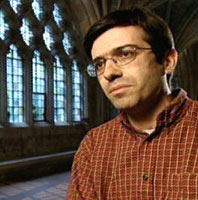In a recent examination of the Archimedes palimpsest, Dr. Reviel Netz discovered what scholars had never realized: that the ancient Greek mathematician had pondered infinity.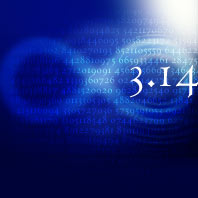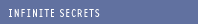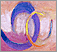Contemplating Infinity Philosophically, the concept remains a mind-bender.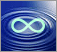Working with Infinity Mathematicians have become increasingly comfortable with the concept.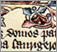Great Surviving Manuscripts Ancient documents offer a tantalizing glimpse of lost cultures.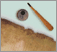The Archimedes Palimpsest Follow the 1,000-year-long journey of the Archimedes manuscript.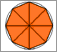Approximating Pi See Archimedes' geometrical approach to estimating pi.Interview conducted and edited by Peter Tyson, editor in chief of NOVA online.